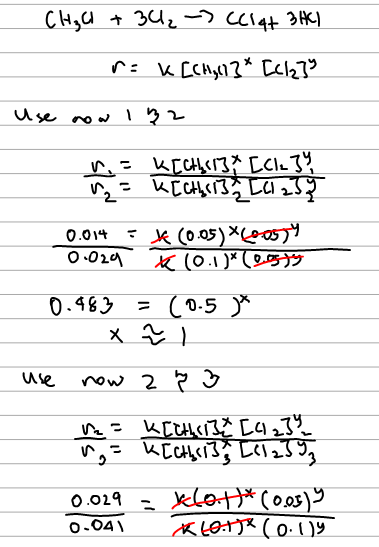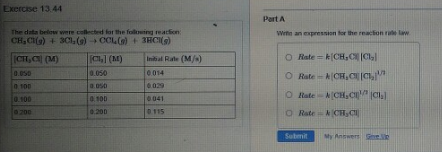# Problem: The data below were collected for the following reaction: CH3Cl (g) + 3Cl2 (g) → CCl4 (g) + 3HCl (g) Write an expression for the reaction rate law. (i) Rate = k[CH3Cl][Cl2](ii) Rate = k[CH3Cl] [Cl2]1/2(iii) Rate = k[CH3Cl]1/2 [Cl2] (iv) Rate = k[CH3Cl]

###### FREE Expert Solution97% (465 ratings)###### Problem Details

The data below were collected for the following reaction:

CH3Cl (g) + 3Cl2 (g) → CCl4 (g) + 3HCl (g)

Write an expression for the reaction rate law.

(i) Rate = k[CH3Cl][Cl2]

(ii) Rate = k[CH3Cl] [Cl2]1/2

(iii) Rate = k[CH3Cl]1/2 [Cl2

(iv) Rate = k[CH3Cl]